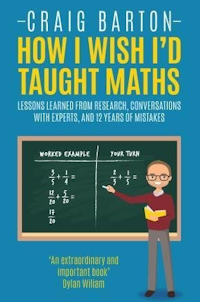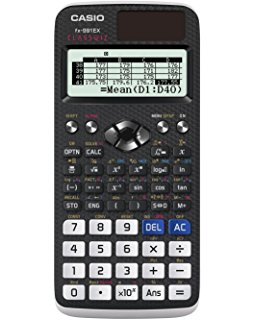Can you use the digits on the left of this clock along with any mathematical operations to equal the digits on the right (also with any mathematical operations)?

## ExamplesWhich times is this possible and which times impossible?

## Alternate activity

Use the digits of the current time to make as many different calculations as possible all with different answers.

All four digits must be used and each must appear once only in each of your calculations.

Next choose a different time that you think will produce more calculations than the present time.

## A Mathematics Lesson Starter Of The Day

Share
• Transum,
•
• The key to making this activity a successful starter is not just considering the current time but focussing on the question 'Which times is this possible and which times impossible?'. The question 'How many times can you find that are possible?' might lead to a list of similar mathematical operations (which is fine for some pupils) while dividing the class into small groups and assigning each group an hour of the day may encourage some more creativity. Let us know if your class make any interesting discoveries.

How did you use this starter? Can you suggest how teachers could present or develop this resource? Do you have any comments? It is always useful to receive feedback and helps make this free resource even more useful for Maths teachers anywhere in the world.

If you don't have the time to provide feedback we'd really appreciate it if you could give this page a score! We are constantly improving and adding to these starters so it would be really helpful to know which ones are most useful. Simply click on a button below:

Excellent, I would like to see more like this
Good, achieved the results I required
Satisfactory
Didn't really capture the interest of the students
Not for me! I wouldn't use this type of activity.

This starter has scored a mean of 3.0 out of 5 based on 306 votes.

Previous Day | This starter is for 14 June | Next Day

 09 01 0x9=0 0x1=0 09 02 0x9=0 0x2=0 09 03 0x9=0 0x3=0 09 04 0x9=0 0x4=0 09 05 0x9=0 0x5=0 09 06 0x9=0 0x6=0 09 07 0x9=0 0x7=0 09 08 0x9=0 0x8=0 09 09 90 90 09 10 0x9=0 1x0=0 09 11 90=1 11=1 09 12 90=1 12=1 09 13 90=1 13=1 09 14 90=1 14=1 09 15 90=1 15=1 09 16 90=1 16=1 09 17 90=1 17=1 09 18 90=1 18=1 09 19 90=1 19=1 09 20 0x9=0 0x2=0 09 21 √9+0=3 2+1=3 09 22 90=1 2÷2=1 09 23 0+9=9 32=9 09 24 √9+0=3, 3!=6 2+4=6 09 25 √9+0=3 5-2=3 09 26 √9+0=3 6÷2=3 09 27 0+9=9 2+7=9 09 28 90=1 82=64, 6+4=10, 1+0=1 09 29 0+9=9 92=81, 8+1=9 09 30 0x9=0 0x3=0

For more ideas see Alan Sturgess' Maths Puzzle Investigation video.

Your access to the majority of the Transum resources continues to be free but you can help support the continued growth of the website by doing your Amazon shopping using the links on this page. Below is an Amazon search box and some items chosen and recommended by Transum Mathematics to get you started.

## Have you read Craig's book yet?

Craig Barton must surely be the voice of Mathematics teachers in the UK. His wonderful podcasts interviewing the industry experts have culminated in this wonderful book. As Craig says: "I genuinely believe I have never taught mathematics better, and my students have never learned more. I just wish I had known all of this twelve years ago..." more...

"How I wish I'd taught Maths" is an extraordinary and important book. Part guide to research, part memoir, part survival handbook, it’s a wonderfully accessible guide to the latest research on teaching mathematics, presented in a disarmingly honest and readable way. I know of no other book that presents as much usable research evidence on the dos and don’ts of mathematics teaching in such a clear and practical way. No matter how long you have been doing it, if you teach mathematics—from primary school to university—this book is for you." Dylan Wiliam, Emeritus Professor of Educational Assessment, UCL.## Casio Classwiz Calculator

There is currently a lot of talk about this new calculator being the best in its price range for use in the Maths classroom. The new ClassWiz features a high-resolution display making it easier to view numerical formulas and symbols but it isn't a graphical calculator as such (it has the capacity to draw graphs on your smart phone or tablet, via a scannable QR code and an app).

As well as basic spreadsheet mode and an equation solving feature you also get the ability to solve quadratic, cubic or quartic polynomial inequalities and the answer is given just as it should be written down, using the correct inequality symbols!

This calculator has a high-performance processor and twice the memory of previous models ensuring speedy operation and superior computational power.more...Teacher, do your students have access to computers?Do they have iPads or Laptops in Lessons? Whether your students each have a TabletPC, a Surface or a Mac, this activity lends itself to eLearning (Engaged Learning).Transum.org/go/?Start=June14

Here is the URL which will take them to a related student activity.

Transum.org/go/?to=fifteen